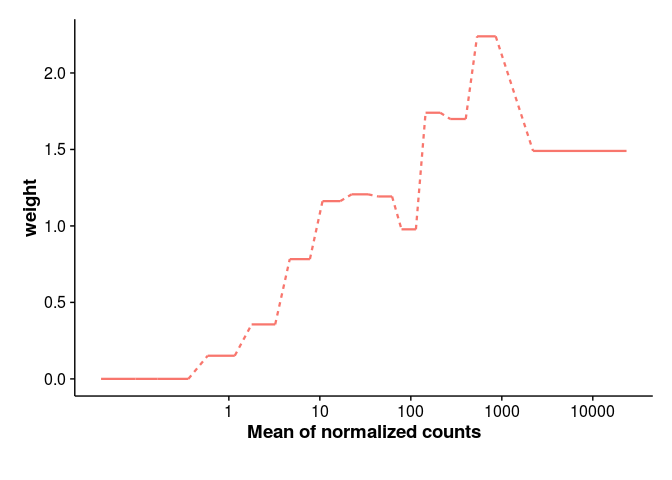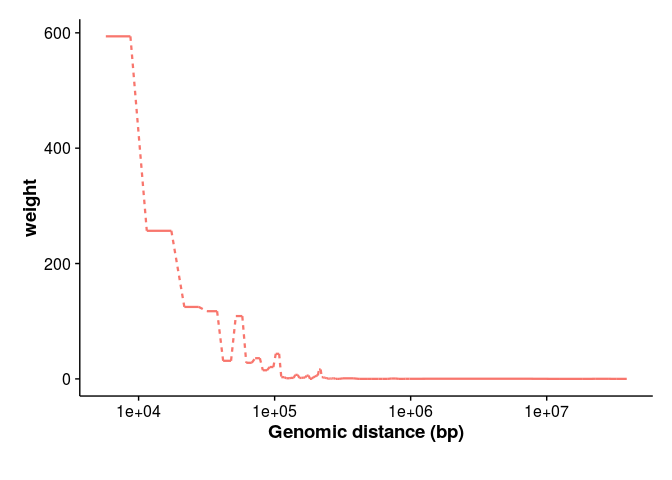# Contents

In this vignette, we want to estimate the weight~covariate function on the RNASeq and eQTL datasets, without using the k-fold strategy. The below plots are mainly useful for presentations.

library("IHW")
library("dplyr")
library("ggplot2")
library("grid")
library("tidyr")
library("cowplot")
library("IHWpaper")
red_col <- scales::hue_pal(h = c(0, 360) + 15, c = 100, l = 65, h.start = 0,direction = 1)(1)

# 1 DESeq2 Bottomly

Load Bottomly dataset, apply DESeq2, then IHW with 1 fold.

bottomly <- analyze_dataset("bottomly")
## estimating size factors
## estimating dispersions
## gene-wise dispersion estimates
## mean-dispersion relationship
## final dispersion estimates
## fitting model and testing
## -- replacing outliers and refitting for 11 genes
## -- DESeq argument 'minReplicatesForReplace' = 7
## -- original counts are preserved in counts(dds)
## estimating dispersions
## fitting model and testing
ihw_res <- ihw(bottomly$pvalue, bottomly$baseMean, 0.1,
nfolds=1, nbins=13,
nfolds_internal=4L, nsplits_internal=5L)
## Using only 1 fold! Only use this if you want to learn the weights, but NEVER for testing!

Do the plotting:

breaks <- IHW:::stratification_breaks(ihw_res)
break_min = min(bottomly$baseMean) breaks_left <- c(break_min,breaks[-length(breaks)]) step_df <- data.frame(weight=weights(ihw_res,levels_only=TRUE), stratum = 1:nlevels(groups_factor(ihw_res))) %>% mutate(baseMean_left = breaks_left[stratum], baseMean_right = breaks[stratum], baseMean_ratio = baseMean_right/baseMean_left , baseMean_left = baseMean_left * baseMean_ratio^.2, baseMean_right = baseMean_right *baseMean_ratio^(-.2)) stratum_fun <- function(df){ stratum <- df$stratum
weight <- df$weight stratum_left <- stratum[stratum != length(stratum)] weight_left <- weight[stratum_left] baseMean_left <- df$baseMean_right[stratum_left]
stratum_right <- stratum[stratum != 1]
weight_right <- weight[stratum_right]
baseMean_right <- df$baseMean_left[stratum_right] data.frame(stratum_left= stratum_left, weight_left= weight_left, stratum_right = stratum_right, weight_right = weight_right, baseMean_left = baseMean_left, baseMean_right = baseMean_right) } connect_df <- step_df %>% do(stratum_fun(.)) %>% mutate(dashed = factor(ifelse(abs(weight_left - weight_right) > 10^(-4) , TRUE, FALSE), levels=c(FALSE,TRUE))) panel_b <- ggplot(step_df, aes(x=baseMean_left, xend=baseMean_right, y=weight, yend=weight)) + geom_segment(size=0.8, color=red_col)+ geom_segment(data= connect_df, aes(x=baseMean_left, xend=baseMean_right, y=weight_left, yend=weight_right, linetype=dashed), size=0.8, color=red_col)+ scale_x_log10(breaks=c(1,10,100,1000,10000))+ xlab("Mean of normalized counts")+ theme(legend.position=c(0.8,0.4)) + theme(plot.margin = unit(c(1, 1, 2, 1), "lines")) + guides(linetype=FALSE) + theme(axis.title = element_text(face="bold") ) panel_bggsave(panel_b, filename="bottomly_1fold_weight_function.pdf", width=7, height=5) # 2 hQTL example Next we load the data for the hQTL example. Here we load the data-frame with the snps, genes and pvalue information. Note that because of memory concerns (the full data-frame is 3 GB) it contains only the p-values below 0.005. However, before doing this reduction we counted the number of hypotheses m_groups in each stratum (strata as defined in group column). Thus we can apply IHW to the reduced dataset, see also the advanced section of the IHW vignette (“Advanced usage: Working with incomplete p-value lists”). hqtl_filt <- system.file("extdata/real_data", "hqtl_pvalue_filtered.Rds", package = "IHWpaper") hqtl_filt <- readRDS(hqtl_filt) m_groups <- attr(hqtl_filt, "m_groups") ihw_qtl_res <- ihw(hqtl_filt$pvalue, as.factor(hqtl_filt$group), 0.1, m_groups = m_groups, nfolds=1L, lambda=Inf) ## Using only 1 fold! Only use this if you want to learn the weights, but NEVER for testing! breaks <- attr(hqtl_filt, "breaks") breaks <- breaks[-1] break_min <- 5000 breaks_left <- c(break_min,breaks[-length(breaks)]) step_df_qtl <- data.frame(weight=weights(ihw_qtl_res,levels_only=TRUE), stratum = 1:nlevels(groups_factor(ihw_qtl_res))) %>% mutate(break_left = breaks_left[stratum], break_right = breaks[stratum], break_ratio = break_right/break_left , break_left =break_left * break_ratio^.2, break_right = break_right *break_ratio^(-.2)) stratum_fun <- function(df){ stratum <- df$stratum
weight <- df$weight stratum_left <- stratum[stratum != length(stratum)] weight_left <- weight[stratum_left] break_left <- df$break_right[stratum_left]
stratum_right <- stratum[stratum != 1]
weight_right <- weight[stratum_right]
break_right <- df\$break_left[stratum_right]
data.frame(stratum_left= stratum_left, weight_left= weight_left,
stratum_right = stratum_right, weight_right = weight_right,
break_left = break_left, break_right = break_right)
}

connecting_df_qtl <- step_df_qtl %>%
do(stratum_fun(.)) %>%
mutate(dashed = factor(ifelse(abs(weight_left - weight_right) > 5,
TRUE, FALSE),
levels=c(FALSE,TRUE)))

panel_f <- ggplot(step_df_qtl, aes(x=break_left, xend=break_right,y=weight, yend=weight)) +
geom_segment(size=0.8, color=red_col)+
geom_segment(data= connecting_df_qtl, aes(x=break_left, xend=break_right,
y=weight_left, yend=weight_right,
linetype=dashed),
size=0.8, color=red_col) +
scale_x_log10(breaks=c(10^4, 10^5,10^6,10^7)) +
xlab("Genomic distance (bp)")+
theme(legend.position=c(0.8,0.4)) +
theme(plot.margin = unit(c(1, 1, 2, 1), "lines"))+
guides(linetype=FALSE) +
theme(axis.title = element_text(face="bold") )

panel_fggsave(panel_f, filename="hqtl_1fold_weight_function.pdf", width=7, height=5)